# Linear representation theory of unitriangular matrix group over a finite field

Jump to: navigation, search
This article gives specific information, namely, linear representation theory, about a family of groups, namely: unitriangular matrix group. This article restricts attention to the case where the underlying ring is a finite field.
View linear representation theory of group families | View other specific information about unitriangular matrix group | View other specific information about group families for rings of the type finite field

This article is about the linear representation theory of the unitriangular matrix group of finite degree$n$ over a finite field. We denote the field size by$q$ and the underlying prime by$p$. Let$r = \log_p q$.

## Summary

Item Value
degrees of irreducible representations over a splitting field (such as$\overline{\mathbb{Q}}$ or$\mathbb{C}$) all are powers of the field size$q$, so the group is a q-power degree group. Moreover, the number of irreducible representations of size$q^d$ is a polynomial of$q$ depending only on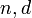$n,d$ and not on$q$.
More information at degrees of irreducible representations of unitriangular matrix group of fixed degree over a finite field are all powers of field size and number of occurrences of each is polynomial function of field size
number of conjugacy classes, which equals number of irreducible representations over a splitting field Polynomial in$q$ of degree$n - 1$, with the coefficients all integers.
More information at number of conjugacy classes in unitriangular matrix group of fixed degree over a finite field is polynomial function of field size
maximum degree of irreducible representation, also the same as lcm of degrees of irreducible representations Seems to be$q^m$ where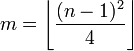$m = \left \lfloor \frac{(n - 1)^2}{4}\right\rfloor$ (the$\lfloor \rfloor$ denotes the floor function, i.e., the greatest integer function) -- proof not available
condition on$n$ for which the group is a finite group in which all partial sum values of squares of degrees of irreducible representations divide the order of the group True for$n = 1,2,3,4$. Not true for$n \ge 5$ (see the #Partial sum values of squares of degrees section).

## Particular cases$n$ Unitriangular matrix group of degree$n$ Linear representation theory page for such a group over a finite field
1 trivial group --
2 additive group of the field, which, for a finite field, is an elementary abelian group --
3 unitriangular matrix group of degree three linear representation theory of unitriangular matrix group of degree three over a finite field
4 unitriangular matrix group of degree four linear representation theory of unitriangular matrix group of degree four over a finite field
5 unitriangular matrix group of degree five linear representation theory of unitriangular matrix group of degree five over a finite field
6 unitriangular matrix group of degree six linear representation theory of unitriangular matrix group of degree six over a finite field

## Degrees of irreducible representations

FACTS TO CHECK AGAINST FOR DEGREES OF IRREDUCIBLE REPRESENTATIONS OVER SPLITTING FIELD:
Divisibility facts: degree of irreducible representation divides group order | degree of irreducible representation divides index of abelian normal subgroup
Size bounds: order of inner automorphism group bounds square of degree of irreducible representation| degree of irreducible representation is bounded by index of abelian subgroup| maximum degree of irreducible representation of group is less than or equal to product of maximum degree of irreducible representation of subgroup and index of subgroup
Cumulative facts: sum of squares of degrees of irreducible representations equals order of group | number of irreducible representations equals number of conjugacy classes | number of one-dimensional representations equals order of abelianization

### Listing of degrees

We denote the field size by$q$ and the underlying prime by$p$. Let$r = \log_p q$.$n$ Total number of irreps equals number of conjugacy classes in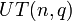$UT(n,q)$ (polynomial of degree$n - 1$ in$q$) Number of irreps of degree 1 Number of irreps of degree$q$ Number of irreps of degree$q^2$ Number of irreps of degree$q^3$ Number of irreps of degree$q^4$ Number of irreps of degree$q^5$ Number of irreps of degree$q^6$
1 1 1
2$q$$q$
3$q^2 + q - 1$$q^2$$q - 1$
4$2q^3 + q^2 - 2q$$q^3$$q^3 - q$
equals$q(q - 1)(q + 1)$$q^2 - q$
equals$q(q - 1)$
5$5q^4 - 5q^2 + 1$$q^4$$2q^4 - q^3 - q^2$
equals$q^2(q - 1)(2q + 1)$$2q^4 - q^3 - 2q^2 + q$
equals$q(q - 1)(q + 1)(2q - 1)$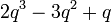$2q^3 - 3q^2 + q$
equals$q(q-1)(2q - 1)$$q^2 - 2q + 1$
equals$(q - 1)^2$
6  ?$q^5$$3q^5- 2q^4 - q^3$
equals$q^3(q - 1)(3q + 1)$
?  ?  ?  ?$q(q - 1)^2$
equals$q^3 - 2q^2 + q$

### Partial sum values of squares of degrees

For visual clarity, we omit cells beyond the maximum degree of irreducible representation. As we see from the table below, each of the unitriangular matrix groups up to degree four is a finite group in which all partial sum values of squares of degrees of irreducible representations divide the order of the group. However, the unitriangular matrix groups of degree five and higher do not have this property.$n$ Order of$UT(n,q)$ equals$q^{\binom{n}{2}}$ Sum of squares of degrees for degree 1 irreps Sum of squares of degrees for irreps of degree dividing$q$ Sum of squares of degrees for irreps of degree dividing$q^2$ Sum of squares of degrees for irreps of degree dividing$q^3$ Sum of squares of degrees for irreps of degree dividing$q^4$ Sum of squares of degrees for irreps of degree dividing$q^5$ Sum of squares of degrees for irreps of degree dividing$q^6$
1 1 1
2$q$$q$
3$q^3$$q^2$$q^3$
4$q^6$$q^3$$q^5$$q^6$
5$q^{10}$$q^4$$2q^6 - q^5$
equals$q^5(2q - 1)$$2q^8 - q^7$
equals$q^7(2q - 1)$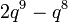$2q^9 - q^8$
equals$q^8(2q - 1)$$q^{10}$
6$q^{15}$$q^5$$3q^7 - 2q^6$
equals$q^6(3q - 2)$
?  ?  ?$2q^{14} - q^{13}$
equals$q^{13}(2q - 1)$$q^{15}$